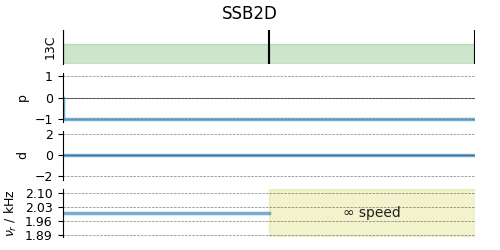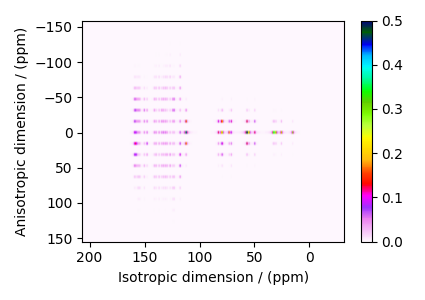# Itraconazole, ¹³C (I=1/2) PASS¶

¹³C (I=1/2) 2D Phase-adjusted spinning sideband (PASS) simulation.

The following is a simulation of a 2D PASS spectrum of itraconazole, a triazole containing drug prescribed for the prevention and treatment of fungal infection. The 2D PASS spectrum is a correlation of finite speed MAS to an infinite speed MAS spectrum. The parameters for the simulation are obtained from Dey et al. 1.

import matplotlib.pyplot as plt

from mrsimulator import Simulator
from mrsimulator.method.lib import SSB2D
from mrsimulator import signal_processor as sp
from mrsimulator.method import SpectralDimension


There are 41 $$^{13}\text{C}$$ single-site spin systems partially describing the NMR parameters of itraconazole. We will import the directly import the spin systems to the Simulator object using the load_spin_systems method.

sim = Simulator()

filename = "https://ssnmr.org/sites/default/files/mrsimulator/itraconazole_13C.mrsys"


Use the SSB2D method to simulate a PASS, MAT, QPASS, QMAT, or any equivalent sideband separation spectrum. Here, we use the method to generate a PASS spectrum.

PASS = SSB2D(
channels=["13C"],
magnetic_flux_density=11.74,
rotor_frequency=2000,
spectral_dimensions=[
SpectralDimension(
count=20 * 4,
spectral_width=2000 * 20,  # value in Hz
label="Anisotropic dimension",
),
SpectralDimension(
count=1024,
spectral_width=3e4,  # value in Hz
reference_offset=1.1e4,  # value in Hz
label="Isotropic dimension",
),
],
)
sim.methods = [PASS]  # add the method.

# A graphical representation of the method object.
plt.figure(figsize=(5, 2.5))
PASS.plot()
plt.show()For 2D spinning sideband simulation, set the number of spinning sidebands in the Simulator.config object to spectral_width/rotor_frequency along the sideband dimension.

sim.config.number_of_sidebands = 20

# run the simulation.
sim.run()


Apply post-simulation processing. Here, we apply a Lorentzian line broadening to the isotropic dimension.

dataset = sim.methods.simulation
processor = sp.SignalProcessor(
operations=[
sp.IFFT(dim_index=0),
sp.apodization.Exponential(FWHM="100 Hz", dim_index=0),
sp.FFT(dim_index=0),
]
)
processed_dataset = processor.apply_operations(dataset=dataset).real
processed_dataset /= processed_dataset.max()


The plot of the simulation.

plt.figure(figsize=(4.25, 3.0))
ax = plt.subplot(projection="csdm")
cb = ax.imshow(processed_dataset, aspect="auto", cmap="gist_ncar_r", vmax=0.5)
plt.colorbar(cb)
ax.invert_xaxis()
ax.invert_yaxis()
plt.tight_layout()
plt.show()1

Dey, K .K, Gayen, S., Ghosh, M., Investigation of the Detailed Internal Structure and Dynamics of Itraconazole by Solid-State NMR Measurements, ACS Omega (2019) 4, 21627. DOI:10.1021/acsomega.9b03558

Total running time of the script: (0 minutes 1.126 seconds)

Gallery generated by Sphinx-Gallery